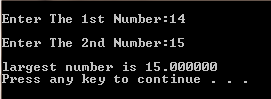# C Program to find the Greatest of the Two Numbers, Without If-Else Statement, Using Math Function

/*In the following program the user will be asked for 2 numbers, then the formula (abs(a+b)/2)+(abs(a-b)/2) will calculate the greatest of the two entered numbers. The Bold text is the C Code here, & the comments are in normal font*/

#include<math.h>   //For abs() function
#include<stdio.h>   //For Standard Input/Output Functions
#include<stdlib.h>  //For system("pause") function
void main()
{
float a,b;
printf("\nEnter The 1st Number:");
scanf("%f",&a);
printf("\nEnter The 2nd Number:");
scanf("%f",&b);

float c=(abs(a+b)/2)+(abs(a-b)/2);

printf("\nlargest number is %f\n",c);
//Printing 2nd number
system("pause");
return 0;
}

ScreenShot:C Program to find the Greatest of the Two Numbers, Without If-Else Statement, Using Math Function